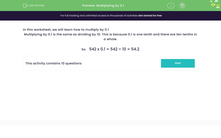# Multiplying by 0.1

In this worksheet, students multiply numbers by 0.1.Key stage:  KS 3

Curriculum topic:   Number

Curriculum subtopic:   Use Four Operations for All Numbers

Difficulty level:#### Worksheet Overview

In this worksheet, we will learn how to multiply by 0.1

Multiplying by 0.1 is the same as dividing by 10. This is because 0.1 is one tenth and there are ten tenths in a whole.

So    542 x 0.1 = 542 ÷ 10 = 54.2

### What is EdPlace?

We're your National Curriculum aligned online education content provider helping each child succeed in English, maths and science from year 1 to GCSE. With an EdPlace account you’ll be able to track and measure progress, helping each child achieve their best. We build confidence and attainment by personalising each child’s learning at a level that suits them.

Get started